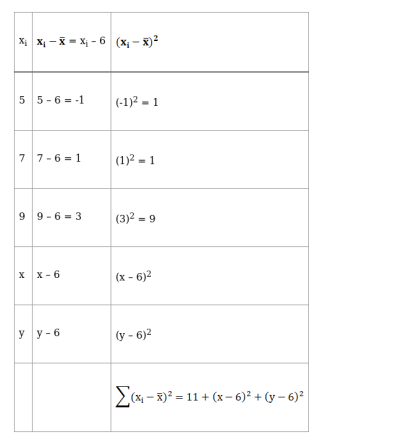# The mean and variance of five observations are 6 and 4 respectively.

Question:

The mean and variance of five observations are 6 and 4 respectively. If three of these are 5, 7 and 9, find the other two observations.

Solution:

Given: Mean of 5 observations = 6

and Variance of 5 observations $=4$

Let the other two observations be $x$ and $y$

$\therefore$, our observations are $5,7,9, \mathrm{x}$ and $\mathrm{y}$

Now, we know that,

$\operatorname{Mean}(\overline{\mathrm{x}})=\frac{\text { Sum of observations }}{\text { Total number of observations }}$

$6=\frac{5+7+9+x+y}{5}$

$\Rightarrow 6 \times 5=21+x+y$

$\Rightarrow 30-21=x+y$

$\Rightarrow 9=x+y$

or $x+y=9 \ldots$ (i)

Also,

Variance = 4

Variance, $\sigma^{2}=\frac{\sum\left(\mathrm{x}_{\mathrm{i}}-\overline{\mathrm{x}}\right)^{2}}{\mathrm{n}}$So Variance, $\sigma^{2}=\frac{\sum\left(\mathrm{x}_{\mathrm{i}}-\overline{\mathrm{x}}\right)^{2}}{\mathrm{n}}$

$4=\frac{11+(x-6)^{2}+(y-6)^{2}}{5}$

$\Rightarrow 20=11+\left(x^{2}+36-12 x\right)+\left(y^{2}+36-12 y\right) \Rightarrow 20-11=x^{2}+36-12 x+y^{2}+36-12 y$

$\Rightarrow 9=x^{2}+y^{2}+72-12(x+y)$

$\Rightarrow x^{2}+y^{2}+72-12(9)-9=0$ [from (i)]

$\Rightarrow x^{2}+y^{2}+63-108=0$

$\Rightarrow x^{2}+y^{2}-45=0$

$\Rightarrow x^{2}+y^{2}=45 \ldots$ (ii)

From eq. (i)

x + y = 9

Squaring both the sides, we get

$(x+y)^{2}=(9)^{2}$

$\Rightarrow x^{2}+y^{2}+2 x y=81$

$\Rightarrow 45+2 x y=81[$ from (ii)]

$\Rightarrow 2 x y=81-45$

$\Rightarrow 2 x y=36$

$\Rightarrow x y=18$

$\Rightarrow \mathrm{x}=\frac{18}{\mathrm{y}}$ …(iii)

Putting the value of x in eq. (i), we get

x + y = 9

$\Rightarrow \frac{18}{y}+y=9$

$\Rightarrow \frac{18+y^{2}}{y}=9$

$\Rightarrow y^{2}+18=9 y$

$\Rightarrow y^{2}-9 y+18=0$

$\Rightarrow y^{2}-6 y-3 y+18=0$

$\Rightarrow y(y-6)-3(y-6)=0$

$\Rightarrow(y-3)(y-6)=0$

$\Rightarrow y-3=0$ and $y-6=0$

$\Rightarrow y=3$ and $y=6$

For y = 3

$\mathrm{x}=\frac{18}{\mathrm{y}}=\frac{18}{3}=6$

Hence, x = 6, y = 3 are the remaining two observations

For y = 6

$x=\frac{18}{y}=\frac{18}{6}=3$

Hence, x = 3, y = 6 are the remaining two observations

Thus, remaining two observations are 3 and 6.